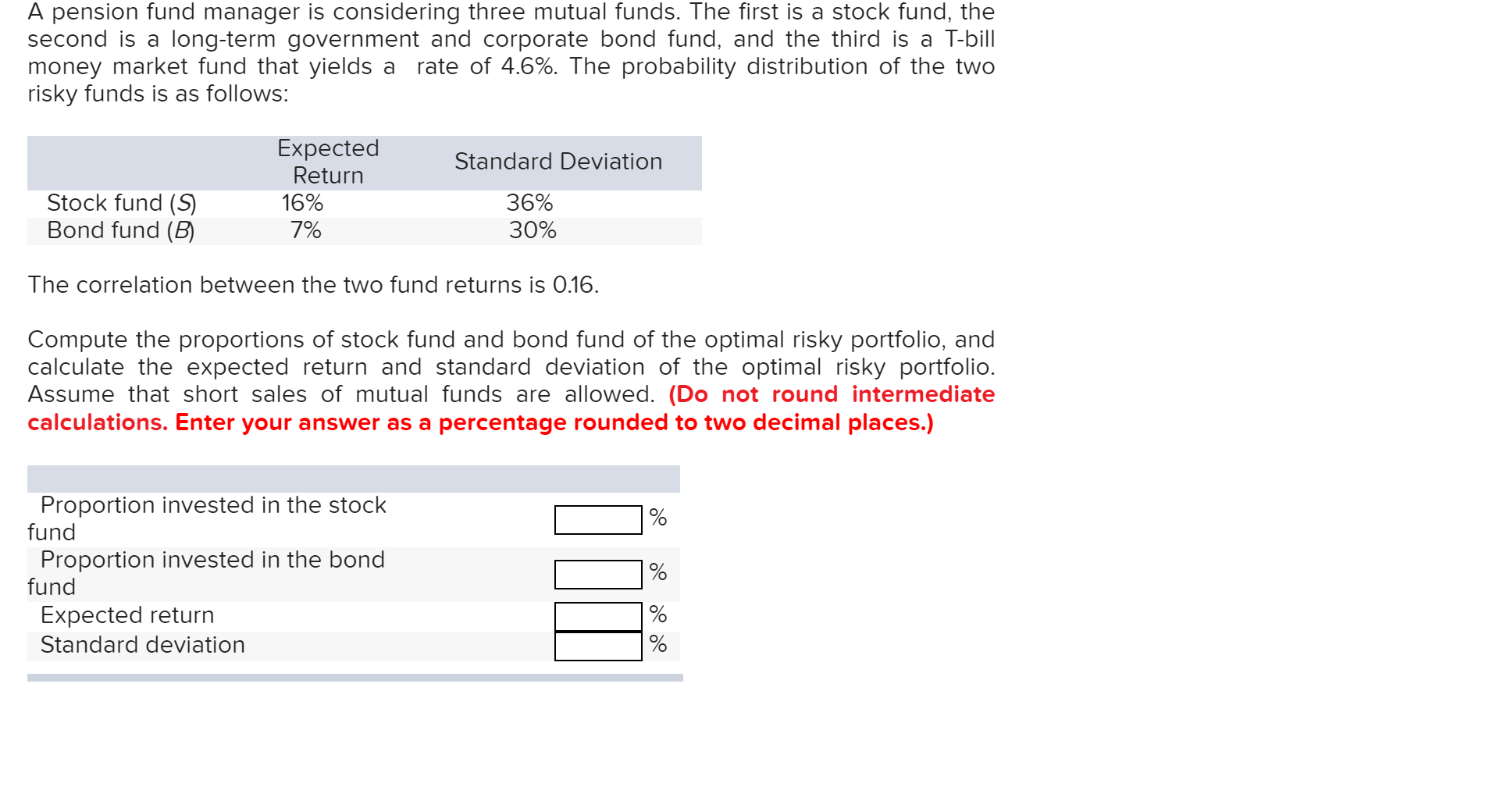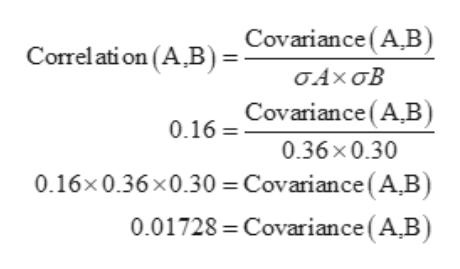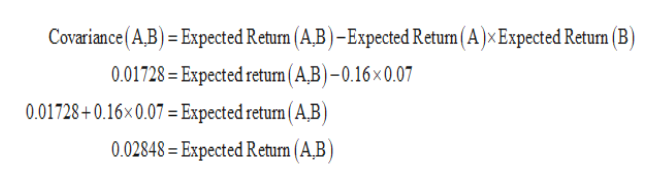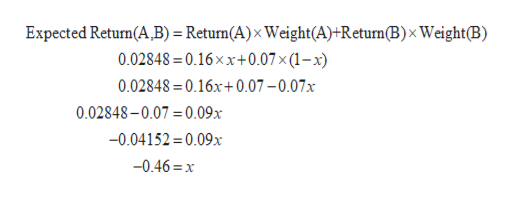A pension fund manager is considering three mutual funds. The first is a stock fund, thelong-term government and corporate bond fund, and the third is a T-billrate of 4.6%. The probability distribution of the twosecond is amoney market fund that yields arisky funds is as follows:ExpectedReturnStandard DeviationStock fund (SBond fund (B36%30%16%7%The correlation between the two fund returns is 0.16.Compute the proportions of stock fund and bond fund of the optimal risky portfolio, andcalculate the expected return and standard deviation of the optimal risky portfolio.Assume that short sales of mutual funds are allowed. (Do not round intermediatecalculations. Enter your answer as a percentage rounded to two decimal places.)Proportion invested in the stockfund%Proportion invested in the bondfund%Expected returnStandard deviation

Questionhelp_outlineImage TranscriptioncloseA pension fund manager is considering three mutual funds. The first is a stock fund, the long-term government and corporate bond fund, and the third is a T-bill rate of 4.6%. The probability distribution of the two second is a money market fund that yields a risky funds is as follows: Expected Return Standard Deviation Stock fund (S Bond fund (B 36% 30% 16% 7% The correlation between the two fund returns is 0.16. Compute the proportions of stock fund and bond fund of the optimal risky portfolio, and calculate the expected return and standard deviation of the optimal risky portfolio. Assume that short sales of mutual funds are allowed. (Do not round intermediate calculations. Enter your answer as a percentage rounded to two decimal places.) Proportion invested in the stock fund % Proportion invested in the bond fund % Expected return Standard deviation fullscreen
Step 1

Let the Stock Fund be Security A and Bond Fund be security B

Calculation of Covariance of security A,B:help_outlineImage TranscriptioncloseCorrelation (A B)=Covariance (A,B) σΑχ σΒ O.16=Covariance (A,B) 0.36x0.30 0.16x0.36x0.30 =Covariance (AB) 0.01728 Covariance (A,B fullscreen
Step 2

Calculation of Expected Return of the portfolio(A,B):help_outlineImage TranscriptioncloseCovariance(A.B) = Expected Return (A,B) -Expected Return ( A ) x Expected Retun (B) 0.01728=Expected returm (A,B)-0.16x 0.07 0.01728+0.16x0.07 = Expected return ( A,B) 0.02848= Expected Return (A,B) fullscreen
Step 3

Calculation of Weights of Stock Fund and bond fund:

Therefore the weight of security B be (1-x)i.e (1-(-0...help_outlineImage TranscriptioncloseExpected Return(A B) = Return(A)x Weight(A)+Return(B) x Weight(B) 0.02848 =0.16xx+0.07 x (1 - x) 0.02848= 0.16x+ 0.07 - 0.07x 0.02848-0.07=0.09x -0.04152 0.09x -0.46 x fullscreen

Want to see the full answer?

See Solution

Want to see this answer and more?

Our solutions are written by experts, many with advanced degrees, and available 24/7

See Solution
Tagged in